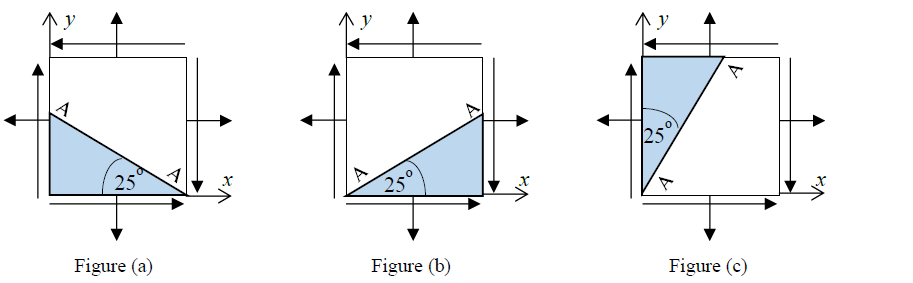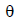# 8.1 Stress Transformation Method of Equations

Choose the best answer in the questions below.
The Poisson’s ratio of the material in questions below is 0.25.Name Email
1.

The angleto find the normal stress on plane AA in Figure (a) using Equation 8.1 is

2.

The angleto find the normal stress on plane AA in Figure (b) using Equation 8.1 is

3.

The angleto find the normal stress on plane AA in Figure (c) using Equation 8.1 is

4.

At a point in plane stress the principal stresses were found to be 22 ksi (T) and 14 ksi (C). The maximum shear stress at the point is

5.

At a point in plane stress the principal stresses were found to be 22 ksi (T) and 14 ksi (T). The maximum shear stress at the point is

6.

At a point in plane strain the principal stresses were found to be 22 ksi (T) and 14 ksi (C). The maximum shear stress at the point  is

7.

At a point in plane strain the principal stresses were found to be 22 ksi (T) and 14 ksi (T). The maximum shear stress at the point is

8.If the other principal stress was 150 MPa (T) then the principal angle 1 is

9.

If the other principal stress was 150 MPa (C) then the principal angle 1 is

10.

If the other principal stress was 75 MPa (T) then the principal angle 1 is